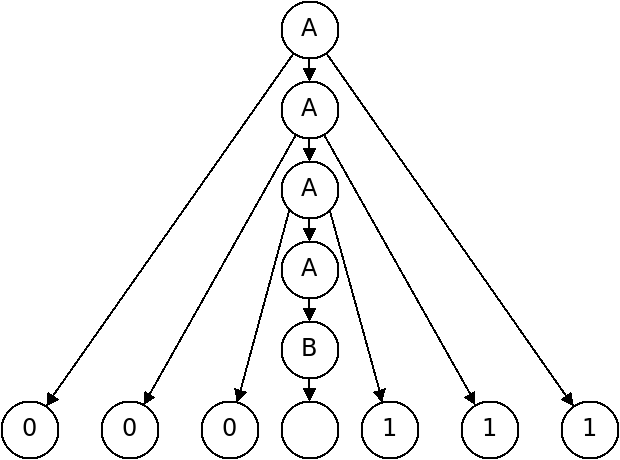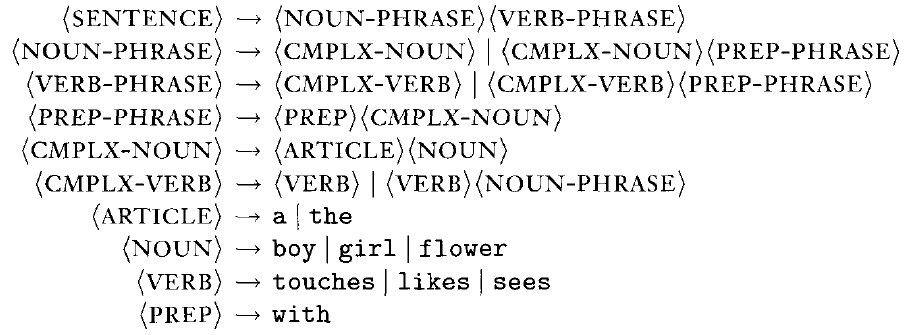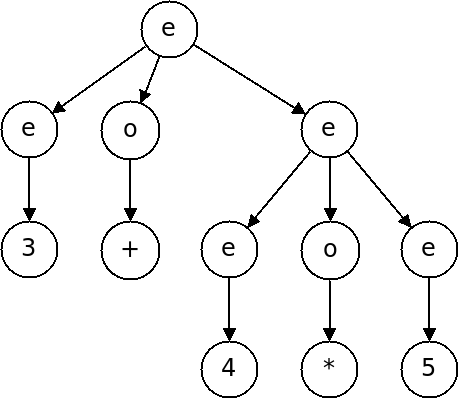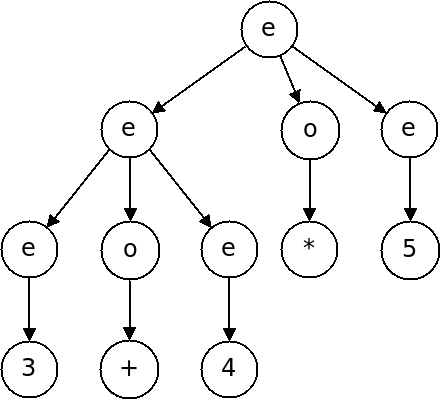# Context-Free Grammars

## Overview

Regular languages are powerful enough to express many things, but we've shown there are languages that are not regular. For example:

$\{0^n1^n \;|\; n \geq 0\}$

Is not a regular language.

This language is, however, a context-free language.

Context-free languages are capable of expressing more complex patterns. Context-free languages include most programming languages.

## Context-Free Grammars

Context-free grammars are a textual way of describing a context-free language.

Below is a context-free grammar for the language above:

$A \rightarrow 0A1$

$A \rightarrow B$

$B \rightarrow \epsilon$

A CFG is comprised of the following:

• Variables

These are symbols that may be substituted for strings of other variables or terminals. $A$ and $B$ are the variables in the above grammar.

• Terminals

Terminals are the symbols that comprise the alphabet of the language we are describing. $0$, $1$, and $\epsilon$ are the terminals above.

• Productions

Productions (or rules) define the substitutions that are permitted in the grammar. A production consists of a left hand side, and arrow, and a right hand side. The left hand side must be a single variable, and the right can be any string of variables and terminals. There are three productions above.

• A start variable

The start variable is the one that we begin the derivation process with. It is typically the left hand side of the first production. $A$ is the start variable above.

## Derivations

Context-free grammars produce strings that belong to the language they describe. The production of a string is called a derivation:

2. Find a variable in the string, and a production with that variable as the left hand side. Replace the variable with the right hand side of the production.
3. Repeat step 2 until no variables remain.

How could we derive "000111" according to the CFG above?




The set of all strings a grammar $G$ can produce is called the language of that grammar and is written as $L(G)$.

## Parse Trees

Another way of depicting a derivation is with a parse tree.

In a parse tree, we start with one node for the start variable - the root of the tree. Each time we follow a production, we make children nodes for each symbol on the right hand side. In the completed parse tree, the terminals are the leaf nodes.

Below is a parse tree for the string "000111":## Language Example

Below is a CFG which describes a fragment of the English language:How can we derive:

• a boy sees
• the girl sees a flower
• a girl with a flower likes the boy

## Formal Definition

A context-free grammar is defined by the following four things:

1. $V$, the finite set of variables.
2. $\Sigma$, the finite set, disjoint from $V$, of terminals.
3. $R$ is a finite set of rules, with each rule having a variable and a string of variables and terminals.
4. $S \in V$ is the start variable.

For convenience we allow multiple productions with the same left hand side to be collapsed. For example:

$A \rightarrow 0A1$

$A \rightarrow B$

$A \rightarrow 0A1 \ |\ B$

When analyzing the grammar, however, this counts as two productions.

## Building Grammars

Like automata or regexes, designing CFGs requires creativity. How can we build a CFG for the language $\{w \;|\; w$ starts and ends with the same symbol$\}$?





## A Grammar for Arithmetic

The following CFG attempts to recognize well-formed arithmetic expressions involving addition, subtraction, multiplication and division:

$\langle expr\rangle \rightarrow \langle expr\rangle \langle op\rangle \langle expr\rangle \ |\ ( \langle expr\rangle ) \ |\ number$

$\langle op\rangle \rightarrow + \ |\ - \ |\ * \ |\ /$

How can we produce the following strings with this grammar:

• $6 * 7$
• $3 + (4 / 7)$
• $1 + 2 + 3$

## Ambiguity

This grammar will distinguish well-formed strings from bad ones, but it is not helpful in capturing the structure of strings because it is ambiguous.

For a string such as $3 + 4 * 5$, there are multiple different ways to derive it:Which of these two is correct?

A grammar is ambiguous if there are two distinct parse trees for the same string, that are both correct in the grammar.

## Ambiguity of Natural Language

The grammar which forms a subset of the English language is below.This grammar is ambiguous as well. Which two ways can we derive:

the girl touches the boy with the flower

Which of these two is correct?

Unlike artificial languages, ambiguity in natural language is something we must accept.

## Disambiguating Arithmetic

There is no set procedure for removing ambiguity from a grammar. We must construct the grammar in such a way so that it forces a derivation to proceed in the way we want.

Below is an improved grammar for arithmetic expressions which forces multiplication and division to have a higher precedence than addition and subtraction:

$\langle expr\rangle \rightarrow \langle expr\rangle \langle addop\rangle \langle term\rangle \ |\ \langle term\rangle$

$\langle term\rangle \rightarrow \langle term\rangle \langle multop\rangle \langle factor\rangle \ |\ \langle factor\rangle$

$\langle factor\rangle \rightarrow number \ |\ ( \langle expr\rangle )$

$\langle addop\rangle \rightarrow + \ |\ -$

$\langle multop\rangle \rightarrow * \ |\ /$

Now what parse tree is produced for $3 + 4 * 5$?

## Applications of Context-Free Grammars

CFG's are widely used to describe artificial languages including arithmetical expressions, but also complete programming languages (here is a grammar for Python). There are even ways of converting a grammar into a program which decides if strings belong to the language or not.